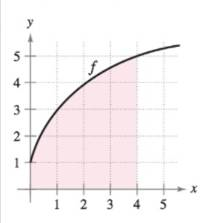Chapter 4.2, Problem 35E

Chapter
Section
Textbook Problem

Using Upper and Lower Sums In Exercises 35 and 36, bound the area of the shaded region by approximating the upper and lower sums. Use rectangles of width 1.To determine

To calculate: Approximate area of the provided region using upper and lower sums.

Explanation

Given: The graph to be considered is:

Formula used:

Formula to calculate the approximate area:

S=i=1nf(Mi)Δx,

Where, Mi are the right endpoints.

And,

s=i=1nf(mi)Δx,

Where, mi are the left endpoints.

Calculation:

The area of region by left endpoints:

s=i=1nf(mi)Δx

Byobserving the graph,

Δx=1, m1=0, m2=1, m3=2, m4=3, f(0)=1, f(1)=3, f(2)=4, and f(3)=4.5.

The area by left endpoints is:

s1(1)+3(1)+4(1)+4

Still sussing out bartleby?

Check out a sample textbook solution.

See a sample solution

The Solution to Your Study Problems

Bartleby provides explanations to thousands of textbook problems written by our experts, many with advanced degrees!

Get Started

Find more solutions based on key concepts